Parameterization is probably the most important topic presented in this course when it comes to developing reusable modeling blocks to flesh out your SIMetrix and SIMPLIS modeling toolkit. A library of properly parameterized subcircuits, whether in sub-schematic or ASCII text form, allows you and your team to spend less time re-implementing the "known" and more time developing your new design IP. In this topic you are introduced to the basics of parameterization, how the netlist preprocessor resolves expressions into real numbers and the scope of parameters.

In this topic:

Key Concepts

This topic addresses the following key concepts:

• Primitive models can be parameterized at the schematic level where they are used by entering the .VAR statement in the F11 window and by adding the expression to the symbol value.
• By default, parameters are local to the hierarchy level.
• Although global parameters can be defined, the recommended method is to pass local parameters down through the hierarchy as explained in  5.1 Passing Parameters Into Subcircuits Using The SIMPLIS_TEMPLATE Property.

What You Will Learn

In this topic, you will learn the following:

• How to parameterize primitive models.
• The scope of local vs. global parameters.
• The unique parameter namespace associated with each schematic component file.

Getting Started

Adding parameters to primitive models, such as resistors, capacitors, and inductors, is easy. You simply enclose the parameter value in curly braces when you enter it in the parameter-editing dialog. For example, the resistor pictured below uses the parameter RLoad for the resistance value: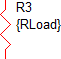Expressions

The text {RLoad} is an expression. In this case, the expression is just the parameter itself; however, you can define the expression as any arbitrary mathematical expression involving parameters and functions as shown in the examples below:

Example Expressions

 Expression Description {Vin} Simple expression involving a single parameter {Vout_nom/Iload} An expression using two parameters, in this case, to calculate a load resistor {C5_val*Gauss(0.05)} An expression using a single parameter and a probability distribution function

Parameters are often defined in the command (F11) window of the schematic using a .VAR statement. In the next exercise, you will learn how parameter values are used in a primitive capacitor model.

Exercise #1: Parameters Used in Primitive Models

1. Open the schematic 5.1_SelfOscillatingConverter_POP.sxsch.
2. In the TL431 compensation network on the schematic, find the capacitor C5 and notice its parameterized value, which is the same as the last expression in the Example Expressions table above.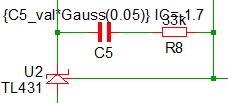3. Press F11 to open the F11 window, and then scroll down to the line which defines the parameter C5_val:
.var C5_val=22n
4. Press F9 to run the simulation.
Result: The simulation executes and the POP waveforms for the converter are displayed on the waveform viewer.
5. From the SIMetrix/SIMPLIS menu bar, select Simulator > Edit Netlist (before preprocess) .
Result: The netlist opens in the netlist editor.
6. To find the text C5_val in the netlist, follow these steps:
1. Type the shortcut Ctrl+F to open the search dialog.
2. Type C5_val in the search box.
3. Click the Find Next button.
Result: The netlist scrolls to line #33 where a .VAR statement defines the parameter. This is the same statement as in the F11 window of the schematic.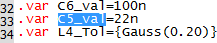4. Click the Find Next button again.
Result: The netlist scrolls to line #47 where the parameter C5_val is used to set the value of the primitive capacitor C5.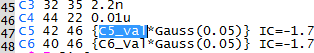Discussion: Exercise #1

Parameters defined in the F11 window can be used to parameterize primitive models.

The capacitor C5 is a primitive capacitor, which is a built-in SIMPLIS model.

• C5 is located on the top-level schematic, 5.1_SelfOscillatingConverter_POP.sxsch, and is parameterized with a .VAR statement in the F11 window of that schematic.
• As a rule, you can define parameters with a .VAR statement in the F11 window of a schematic and then use these parameters in expressions on any symbol placed on the same schematic.

Model Parameters Must be Stored on a Symbol Property

In the 3.0.1 What Happens When You Press F9? topic, you learned how the netlisting process works. In that topic you learned how the netlister substitutes symbol properties in the SIMPLIS_TEMPLATE or TEMPLATE property to generate the netlist entry for a symbol.

The resulting netlist instantiation line defines the electrical device used in the simulation. The instantiation line for the capacitor C5 is as follows:

C5 42 46 {C5_val*Gauss(0.05)} IC=-1.7

The capacitor value is stored on the VALUE symbol property which includes both the parameter expression and the initial condition: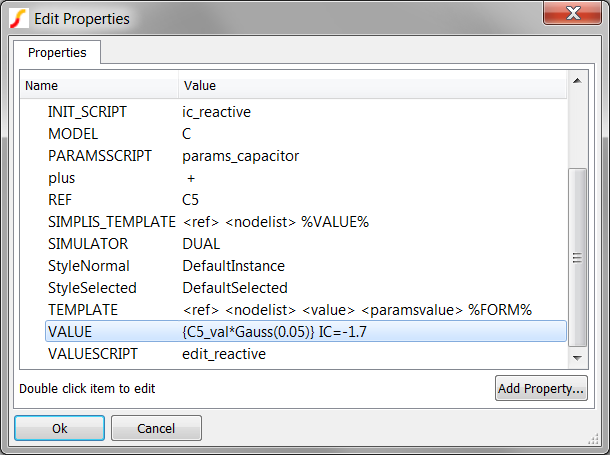The point here is that although the parameter value is defined in the F11 window, the expression containing the parameter must be stored on the VALUE symbol property so that the netlist entry can be generated. As the netlister processes each symbol, it does not know the parameter value is defined in the F11 window. Instead, the netlister simply substitutes the VALUE symbol property into the SIMPLIS_TEMPLATE in place of the text %VALUE%.

The capacitor in this example stores multiple electrical parameters on a single symbol property. This is the single property method and is covered in the Single-Property Method topic. One of the other ways to store parameters is the Multi-Property Method, which stores each electrical parameter on a single symbol property.

Scope of Parameters

By default, parameters are local to the schematic where the parameter is defined. Separate files for each schematic create multiple hierarchy levels. At any schematic level, all parameters defined in the F11 window of the schematic can be used on symbols located on that schematic. Parameters defined in one schematic cannot be used in other schematics unless the parameter is passed down through the symbol or unless the parameter is global. This allows the same parameter name to be used in different schematic components with different values.

The next exercise explores the scope of parameters.

Exercise #2: Parameters are Local to the Hierarchy Level

1. In the opened schematic, 5.1_SelfOscillatingConverter_POP.sxsch, select the optocoupler U1.
2. Type Ctrl+E to descend into the schematic of the optocoupler U1.
3. Press F11 to open the F11 window for the schematic.
Result: The F11 window opens and displays a single .VAR statement:
.VAR CTR=2
4. To comment out the .VAR statement, type a single asterisk (*) in front of the .VAR CTR=2 statement.
Result: The F11 window content is now as follows:
*.VAR CTR=2
5. Select the top level schematic tab: 5.1_SelfOscillatingConverter_POP.sxsch.
6. In the F11 window, locate the same .VAR statement which is the second to last statement in the F11 window.
*.VAR CTR=2
7. To uncomment the .VAR statement, delete the asterisk (*).
Result: The F11 window of the top level schematic now has the .VAR statement:
.VAR CTR=2
8. Press F9 to run the simulation.
****************************************
<<<<<<<< Error Message ID: 1038 >>>>>>>>
input file C:\Training\Module_5_Examples\SIMPLIS_Data/5.1_SelfOscillatingConverter_POP.deck, line 172:
F1 9 6 VF1\$TP_CCCS {CTR}

A number to represent the value
for `proportionality constant' is expected at the
location where `{CTR}' occupies.

Discussion: Exercise #2

In this exercise, you attempted to use the parameter CTR in a hierarchical level other than the top level where CTR is defined. Since the parameters defined at each hierarchy level are independent of each other, the CTR parameter is undefined at the optocoupler level and an error is generated. In the next exercise, you will use the .GLOBALVAR statement to make CTR a global parameter.

Exercise #3: Global Parameters

1. In the F11 window of the top level schematic, comment out the .VAR CTR=2 and uncomment the .GLOBALVAR CTR=2 line.
Result: The F11 window appears as follows:
*.VAR CTR=2
.GLOBALVAR CTR=2
2. Press F9 to run the simulation.
Result: The simulation now runs successfully as expected.

Discussion: Exercise #3

When you define a parameter using the .GLOBALVAR statement, that parameter is available at that hierarchy level and at all lower levels in the hierarchy. This means that the parameter CTR is available at both the top level schematic and at the optocoupler hierarchy level. While this works, there are real and significant dangers of using .GLOBALVAR to parameterize the model.

• Every optocoupler schematic component in the design will have the same CTR parameter. There is no way to change this parameter when a .GLOBALVAR statement is used to define its value.
• If the optocoupler is placed on a new schematic, the CTR parameter might already be defined. This "namespace collision" is exactly what you want to avoid when parameterizing models.
• Someone unfamiliar with the design will not understand where the parameter CTR is defined since CTR is not defined anywhere on the Gen_Opto.sxcmp schematic.
• The Gen_Opto.sxcmp schematic component is not a stand-alone entity - it requires a globally defined parameter. This hinders modularity and portability.

In topic 5.1 Passing Parameters Into Subcircuits Using The SIMPLIS_TEMPLATE Property, you will learn the recommended way to pass parameters into subcircuits.

Conclusions and Key Points to Remember

• Primitive models can be parameterized at the schematic level where they are used by entering the .VAR statement in the F11 window and by adding the expression to the symbol value.
• By default, parameters are local to the hierarchy level.
• Although global parameters can be defined, the recommended method is to pass local parameters down through the hierarchy as explained in  5.1 Passing Parameters Into Subcircuits Using The SIMPLIS_TEMPLATE Property.